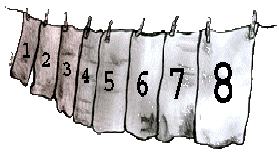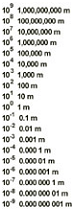Formal Education
Informal Education
Education Collaborations
Web Shortcuts
Modeling the Electromagnetic Spectrum

#### The Exponential ClotheslineMany students have difficulty expressing large numbers using exponential notation. The exponential clothesline helps to pre-assess student understanding of exponents by providing a visual representation of exponential notation (powers of ten). The Exponential Clothesline Conversion Table will also pre-assess and refresh student understanding of fractions and decimals.

Provide each group of students with the following materials:

One 5-meter piece of clothesline (or string)
Fourteen (14) clothespins (or paper clips)
Fourteen (14) index cards with the following numbers identified as follows:
0 written in red
1, 2, and 3, in blue
101, 102, 103, 106, 109, and 1012 in green
10-1, 10-2, 10-3, and 10-4 in black

Numbers written in black on different colored index cards are more visual, and any incorrectly placed numbers are immediately recognizable in a large classroom. The Number and Exponent Cards described above are also available in pdf format for you to download.

Depending on your group, you may wish to provide some larger (or smaller) exponents or include a set of numbers with exponents such as 2 x 100, 2 x 101, 2 x 102 and 2 x 10-2.

Give each group of students the clothesline, clothes pins, and a set of numbered index cards. Randomly distribute the cards as evenly as possible within each group. Have the students string the clothesline, and ask the student in each group with the number 0 to attach that index card approximately one-sixth of the way from the left end of the clothesline. The Students with the number 1 should attach it 25-cm to the right of the number 0.

Explain to the students that their clothesline represents a number line and that they are going to add whole numbers and numbers expressed in scientific notation to the number line. Ask the students who have numbers 2 and 3 in each group to place their numbers on the clothesline. Most students will correctly place whole numbers on the number line. Give the students the task of placing the remaining numbers on the number line in their correct locations. [It is important to explain to your students that this number line is not to scale.] Most groups will discuss and accurately place 101, 102, 103, 106, 109, and 1012 and other higher powers of ten; however when they begin to place the negative exponents on the clothesline, most students will place them to the left of 0, which is a common mistake.The negative exponents actually fall between 0 and 1. Encourage the students to make changes if they think any of the numbers are not in the correct order. If any of the groups think that their number lines are correct and there are still numbers placed out of sequence, hand out the Exponential Clothesline Conversion Table. The purpose of the conversions is to express the exponents as whole numbers, fractions and decimals. After the final conversion of the exponents to decimals, students who have the negative exponents incorrectly placed usually begin to see that negative exponents are still greater than 0 and rearrange their number lines accordingly.

Use the completed Exponential Clothesline Conversion Table to enter into a discussion about what scientific notation is and why it is useful.

The Exponential Clothesline Conversion Table

Modeling the Electromagnetic Spectrum: Middle School
Modeling the Electromagnetic Spectrum: High School